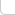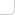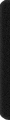# 某岛

… : "…アッカリ～ン . .. . " .. .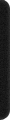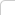August 27, 2011

# SPOJ 9392. Play With Sequence

（由于被 fgd BS 了英文。。我把题目背景都删了。。）

### Brief description :

You are given a sequence A, A,…, A[N]. On this sequence you have to apply M operations: Add all the elements whose value are in range [l, r] by d or, ask for a query how many element are in range [l, r].
( 1≤ N ≤ 250,000, M ≤ 50,000), |A[i]| ≤ 1,000,000,000 .)

### Analysis :

（嘛。。题目出成这样我也没有什么可辩解的了。。
。。开始觉得这是数据结构 S 级神题的。。）

。。。这是这个问题的难点所在。。。下面对 键值处在某个范围的数整题加上某个数 这个操作进行进一步的观察。。

1. 选出的序列，在操作的前后相对顺序不发生改变。
2. 在任意时刻，如果两个数额键值相同，那么今后，它们会一直作为一个整体存在。

….

（一个容易出错的地方是，虽然我保证中间的数据也不会爆 int32，但是在处理 delta 的时候可能会爆。。所以如果想保持主要的结构都使用 int32 的话这里要特判一下。。）

。。但是看上去似乎（对于随机生成的数据）这种情况不会出现 。。

/theta = sqrt Sigma i, j sqr(Ai – Aj)

（于是，重新考察前面的性质2，虽然说要中间恰好相等可能不太会出现。

1. 数列的离散程度下降，同时，块数增多。
2. Nothing happend (什么都没有发生)。。

（我以后会继续考虑这个问题的。。如果有么进展的话会及时更新。。）

P = sqrt(n)
O( (M / log P) N log P) = O(NM)..
P 是块数上限。。 M / log P 是重构频率。。（= =。。。。）

（然后还会 很慢很慢 的 向 O(1) 收敛 。。考虑所有数的值都一样的时候。。大概随机情况下要进行一个天文数字次才可能发生吧。哎。。要会一点概率论就好了啊。。。。。）

```struct Link{
int a, b, d;

Link(int _a, int _b, int _d):a(_a), b(_b), d(_d){};
void Split();
int Stat();
} L[N]; int sz;
```

。。。

。这个 方法在 SPOJ 可以跑 14.54 s。。。

```/* .................................................................................................................................. */

const int N = 250010, M = 1010, C = 1000000000;
int A[N]; char cmd; int cnt;
int n, m, a, b, l, r, d; int *_a, *_b;

int a, b, d;

Link(int _a, int _b, int _d):a(_a), b(_b), d(_d){};
void Split();
int Stat();
} L[N]; int sz;

#define FIX(x) int(x < -C ? -C : x > C ? C : x)
#define GPS _a = lower_bound(A + a, A + b, FIX((LL)::l - d)), _b = upper_bound(A + a, A + b, FIX((LL)::r - d))

GPS, ::a = _a - A, ::b = _b - A;
if (::a == ::b || b < ::a || ::b < a) return;
int aa = max(::a, a), bb = min(::b, b);

if (a < aa) L[sz++] = Link(a, aa, d);
if (bb < b) L[sz++] = Link(bb, b, d);
a = aa, b = bb, d += ::d;
}

GPS; return _b - _a;
}

void Rebuild(){
REP(i, sz) FOR(j, L[i].a, L[i].b) A[j] += L[i].d;
sort(A, A+n), L = Link(0, n, 0), sz = 1;
}

/*
void Patch(){

REP(i, sz){
FOR(j, L[i].a, L[i].b)
cout << A[j] + L[i].d << " ";
}
cout << endl;
}*/

int main(){

//freopen("in.txt", "r", stdin);
//freopen("out.txt", "w", stdout);

RD(n, m); REP(i, n) RD(A[i]); sort(A, A+n);
L = Link(0, n, 0), sz = 1;
int P = sqrt(n) / 11;

char cmd; REP(j, m){
scanf(" %c", &cmd);

if (cmd == 'C'){
RD(l, r, d); REP_C(i, sz) L[i].Split();
if (sz > P) Rebuild();
//Patch();
}
else {
RD(l, r); cnt = 0; REP(i, sz) cnt += L[i].Stat();
OT(cnt);
}

}
}
```

。如何构造一个数据可以杀掉所有 std 呢。。
（、这个问题留给读者思考。。）